# 優的民宿 YUDO Excellent B&B

2,800

#### 臨近：

飯店位置： 冬山鄉 客房數： 0

## 選擇專案房型及間數

更改 優的民宿 的入住日期

#### 更改 優的民宿 的空房情形及價格

入住日期 停留夜數 退房日期
•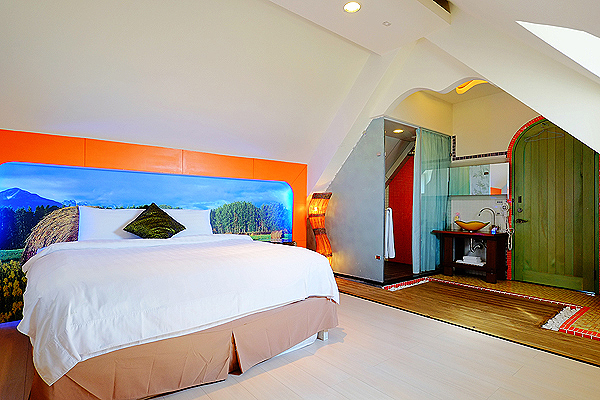環境
•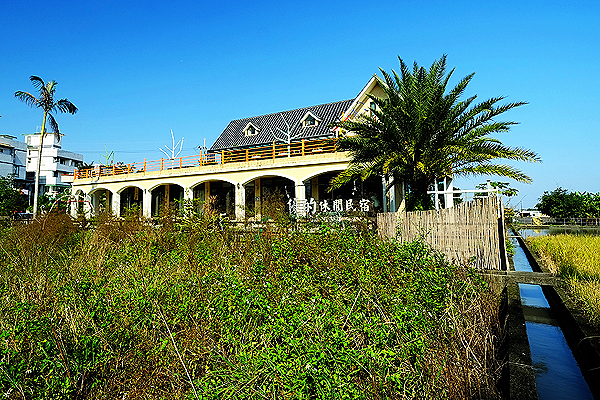環境
•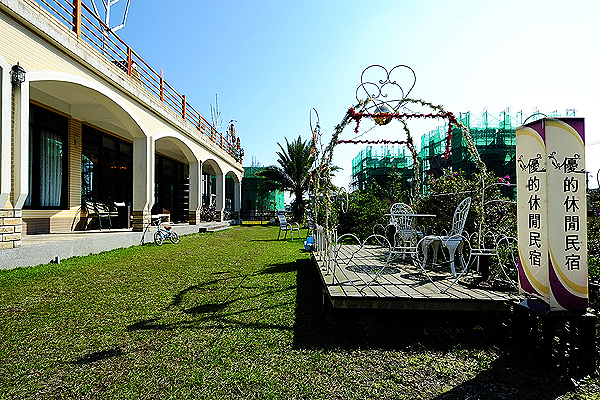環境
•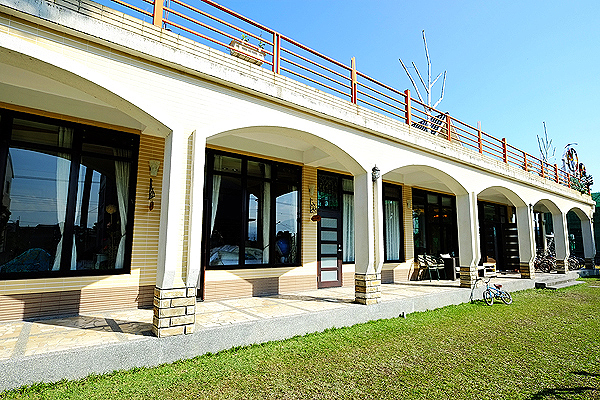環境
•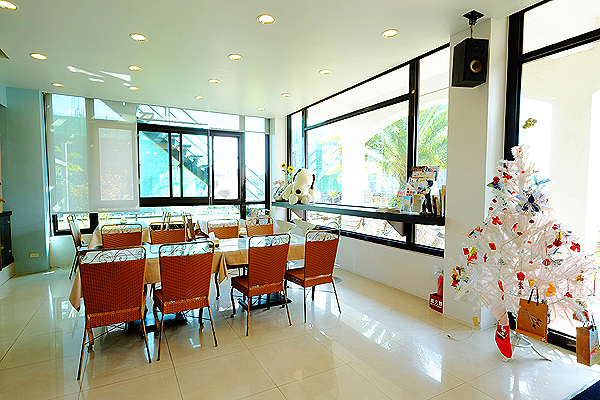環境
•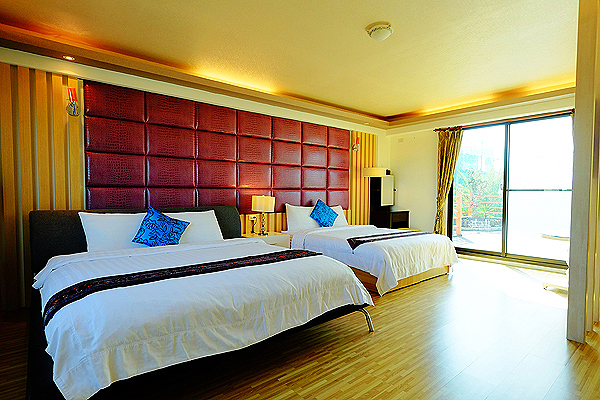環境
•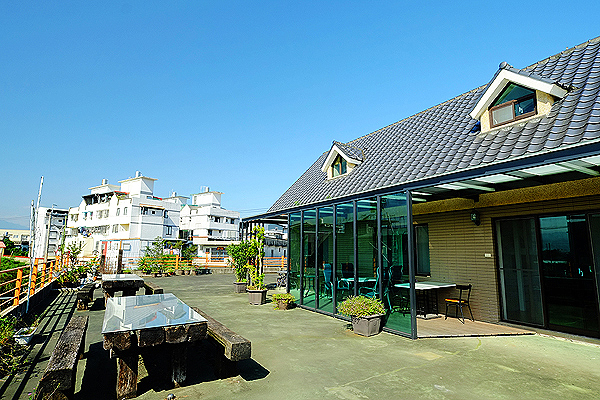環境
•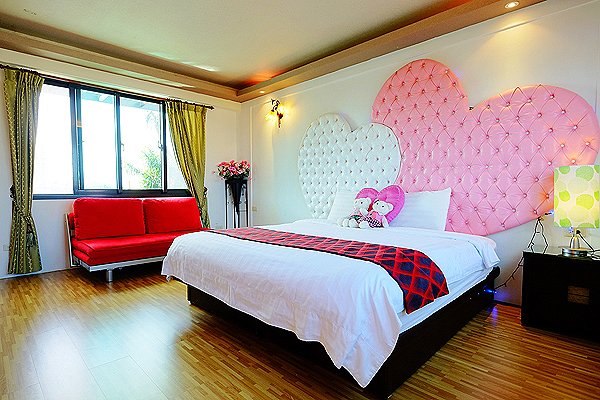環境
•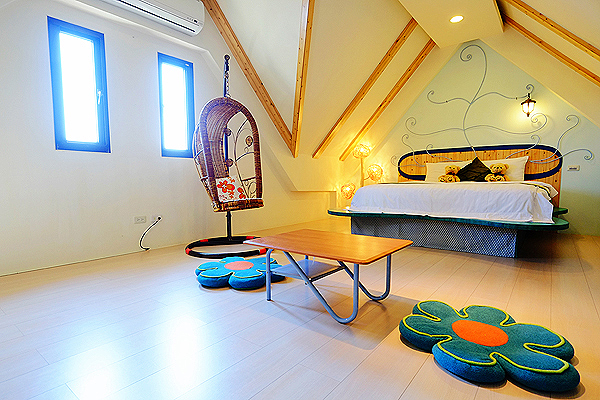環境
•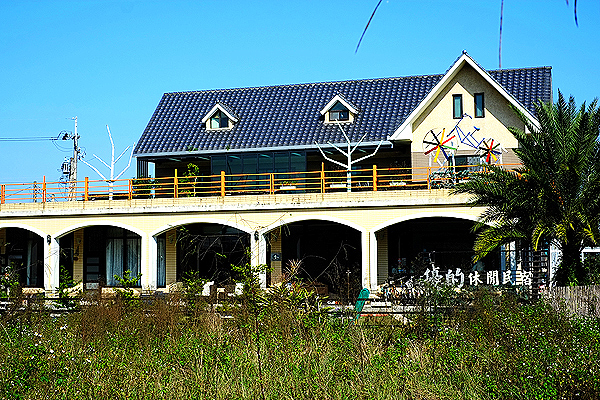環境
•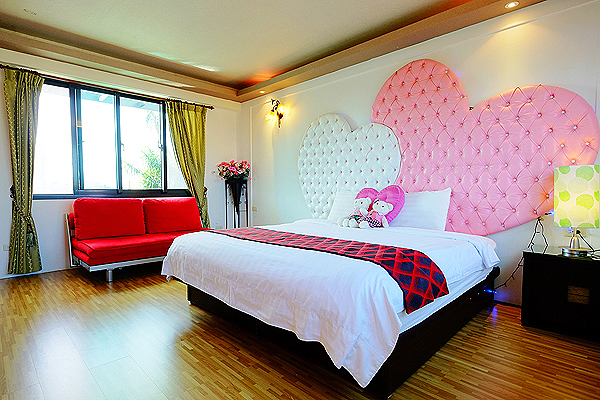客房
•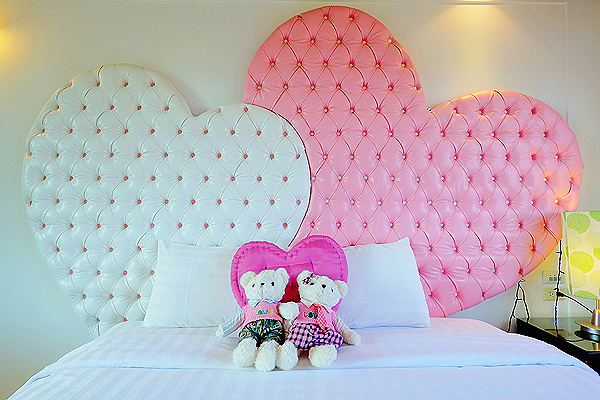客房
•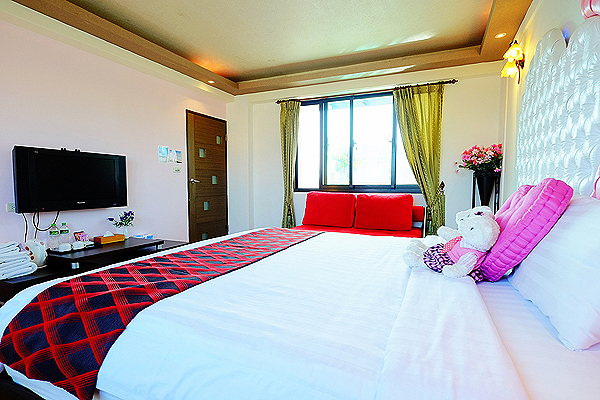客房
•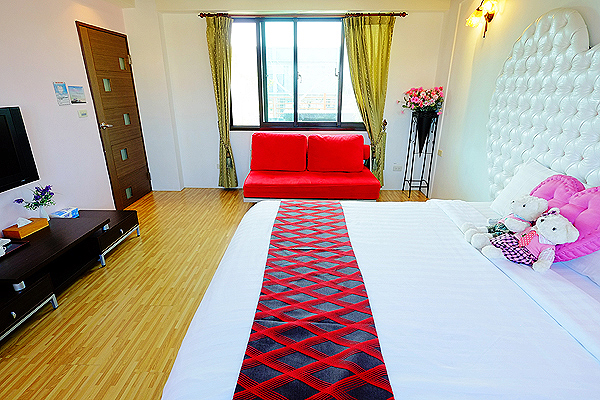客房
•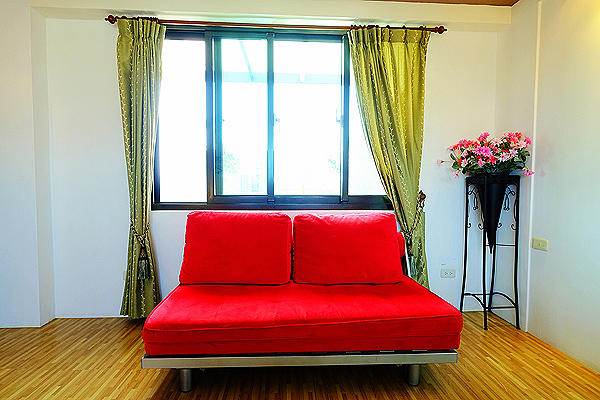客房
•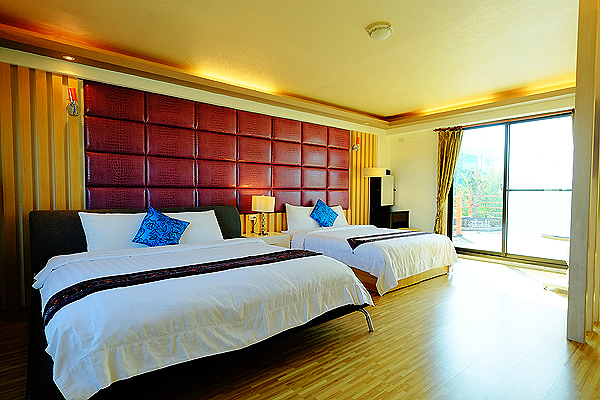客房
•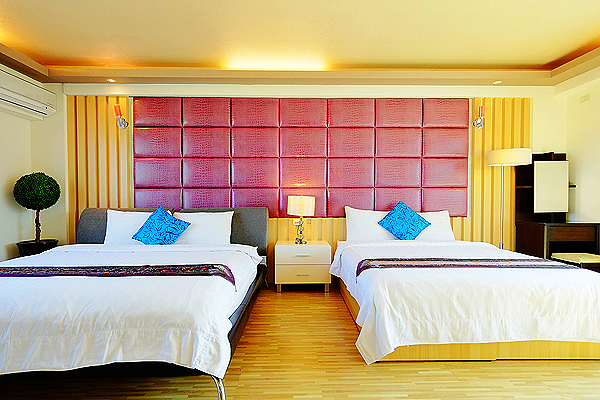客房
•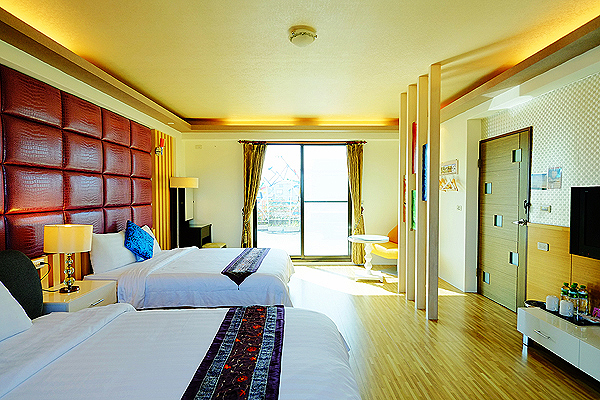客房
•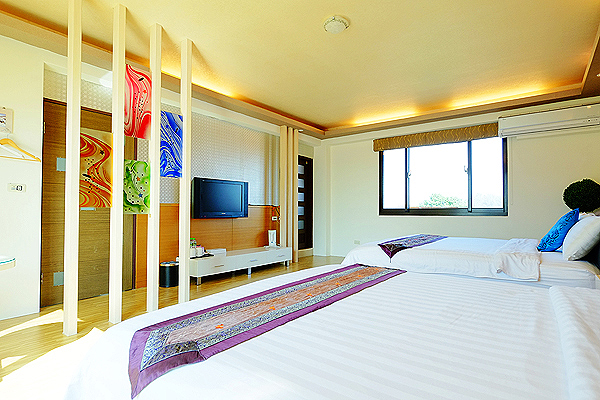客房
•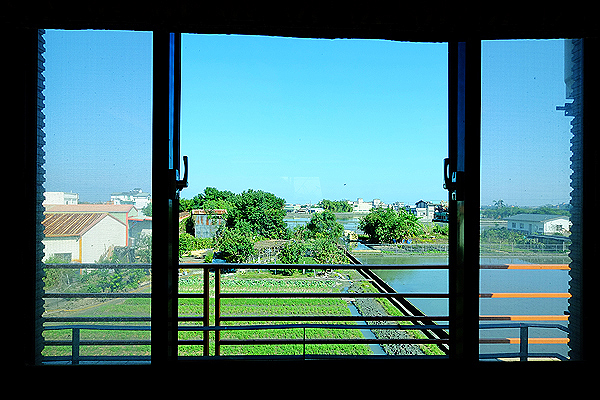客房
•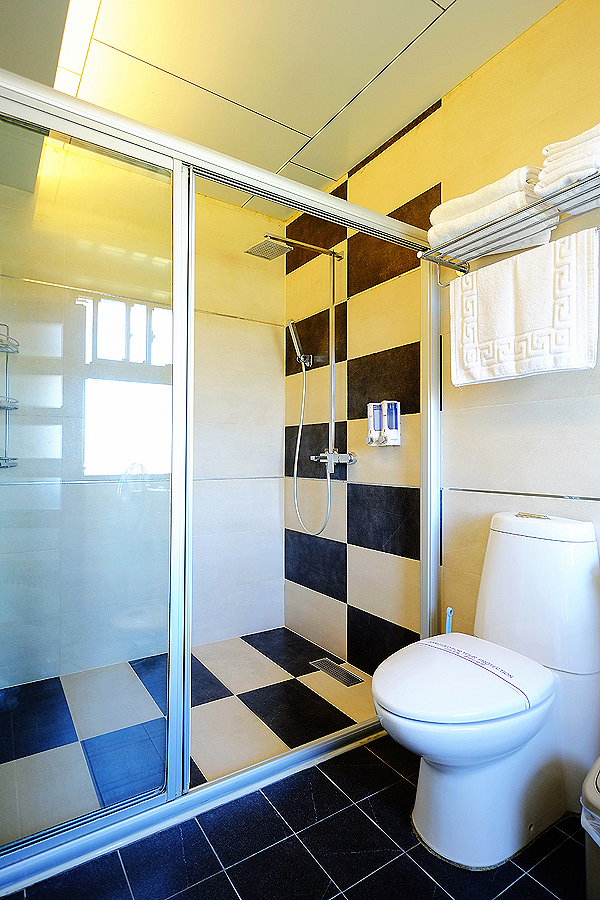客房
•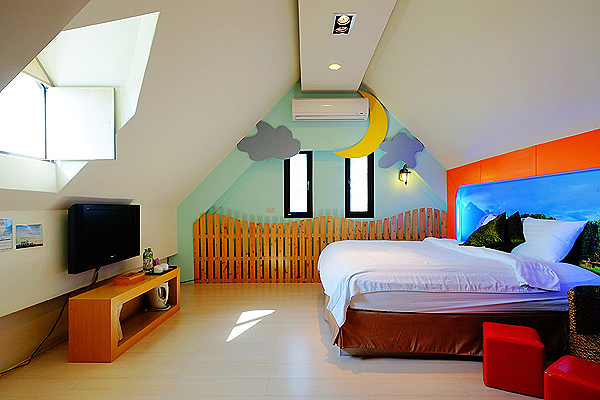客房
•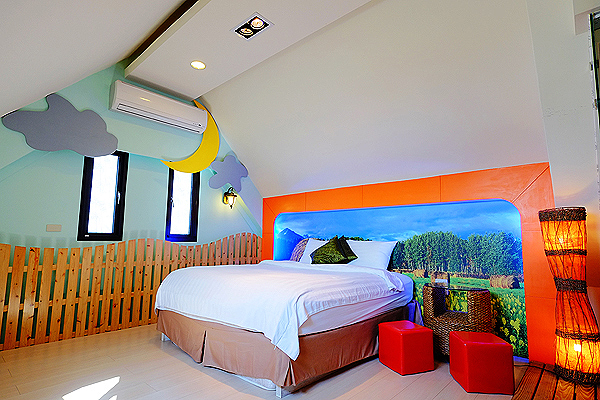客房
•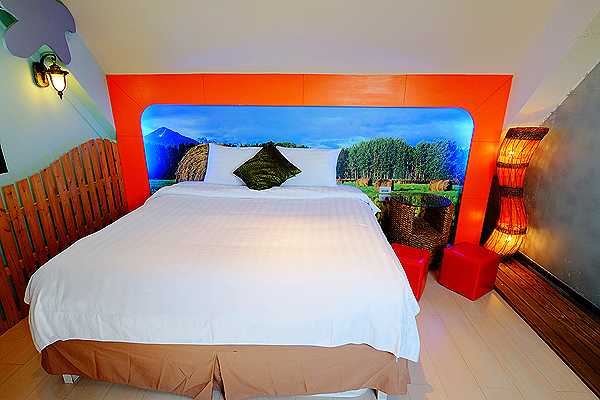客房
•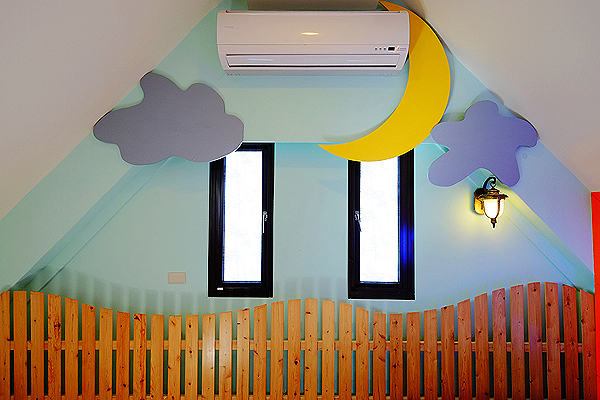客房
•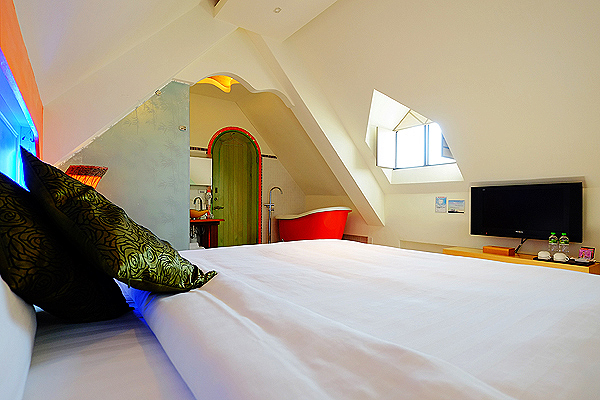客房
•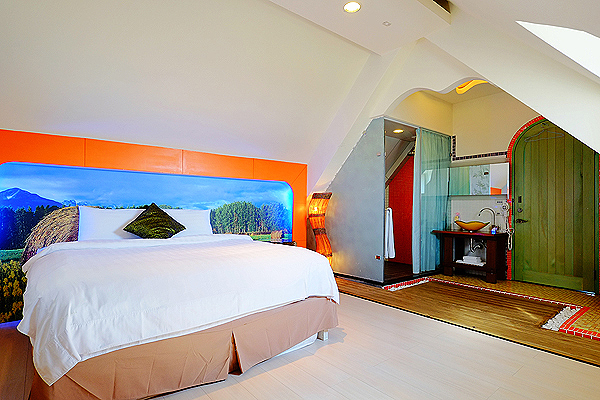客房
•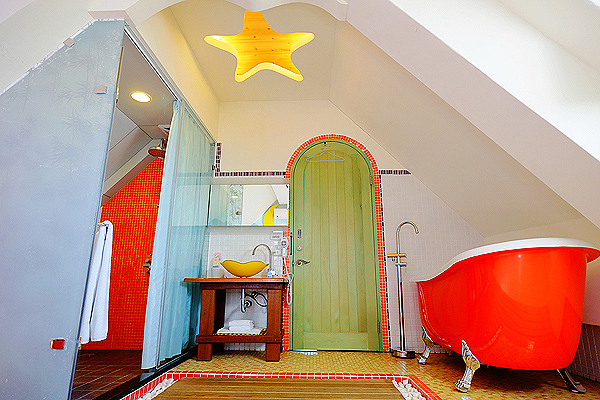客房
•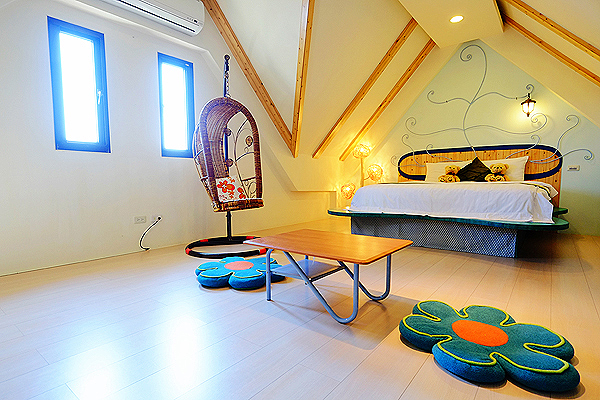客房
•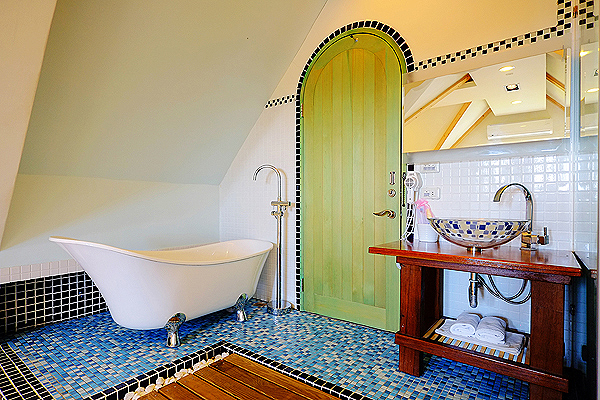客房
•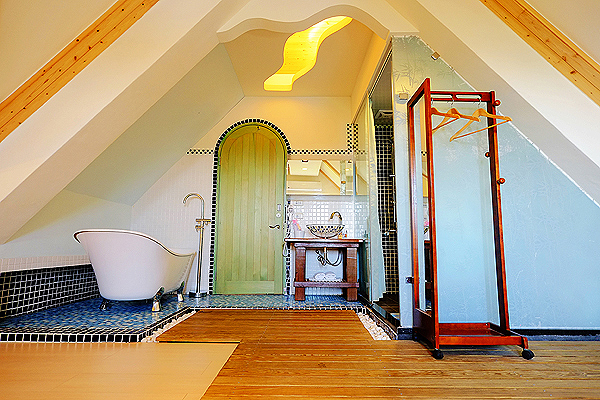客房
•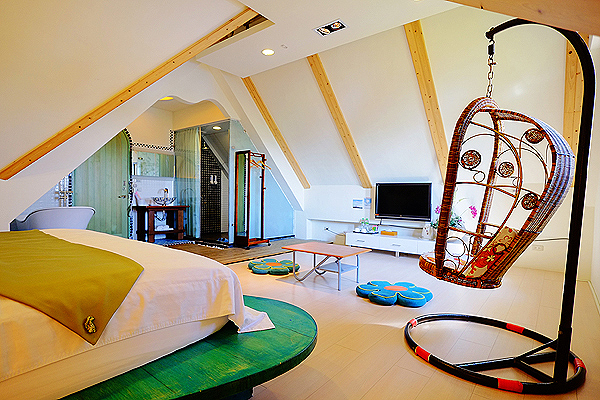客房
•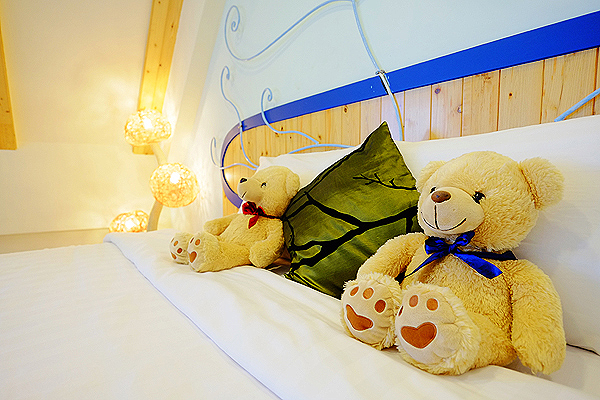客房
•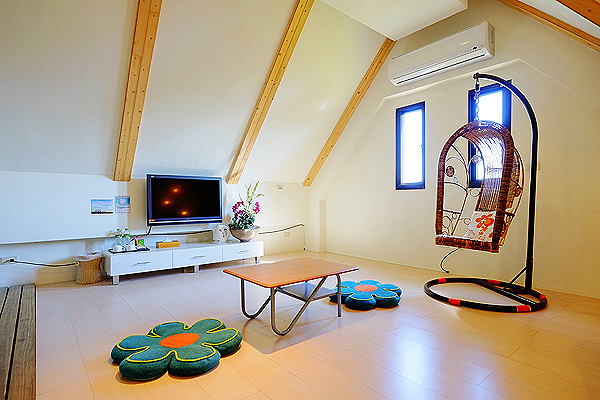客房
•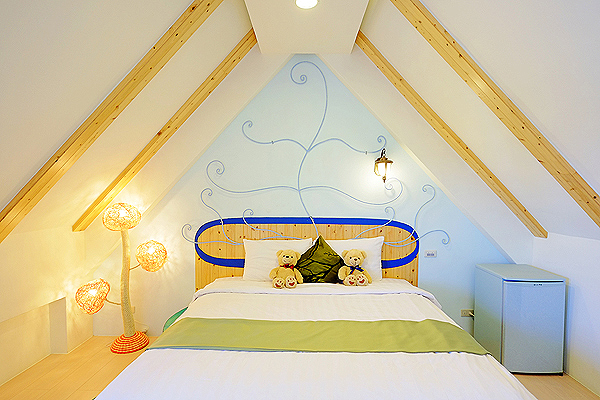客房
•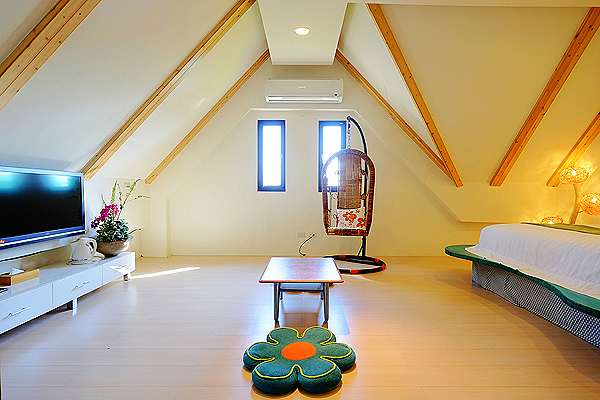客房
•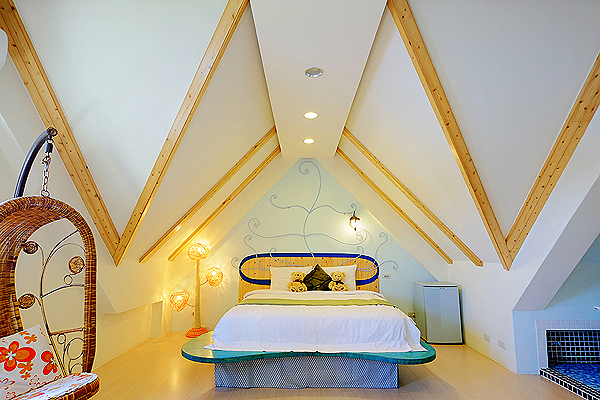客房
•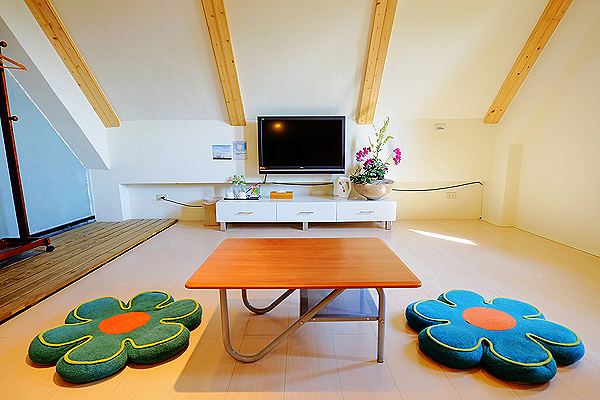客房
•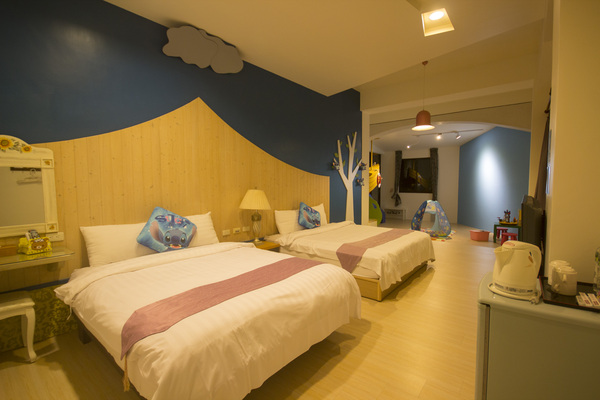客房
•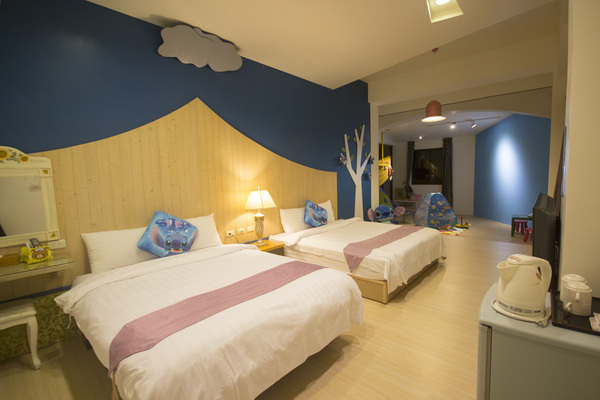客房
•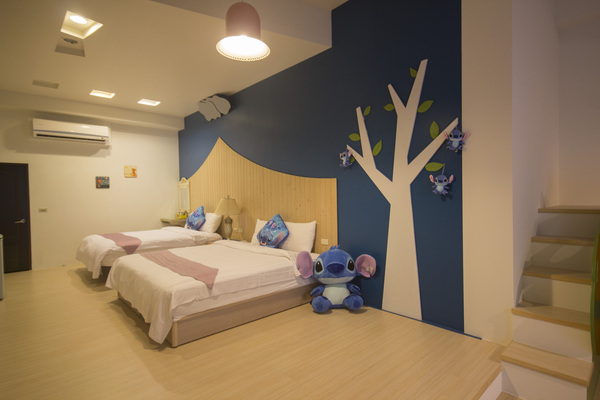客房
•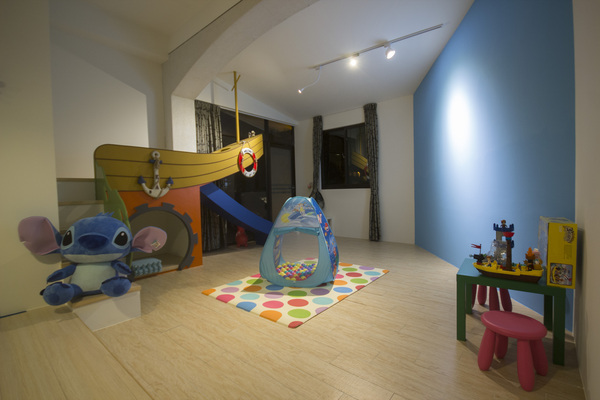客房
•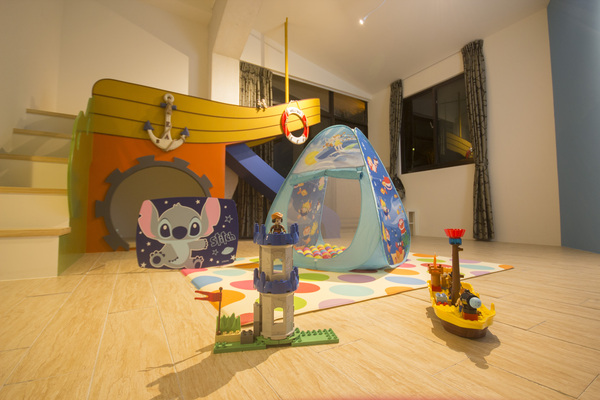客房
•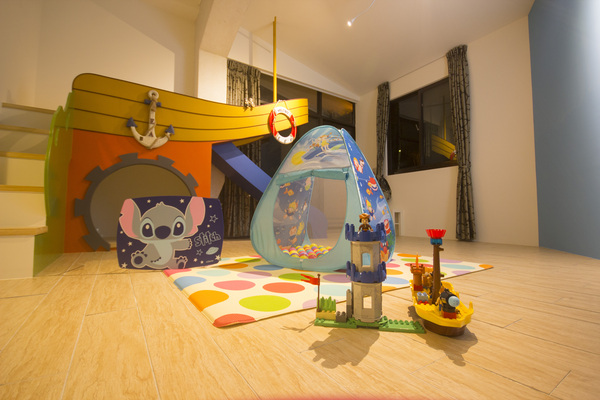客房
•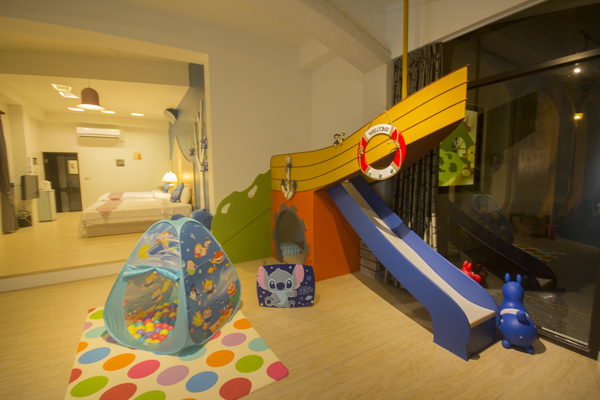客房
•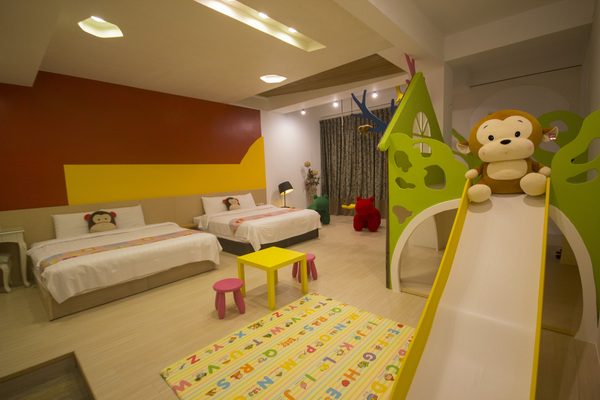客房
•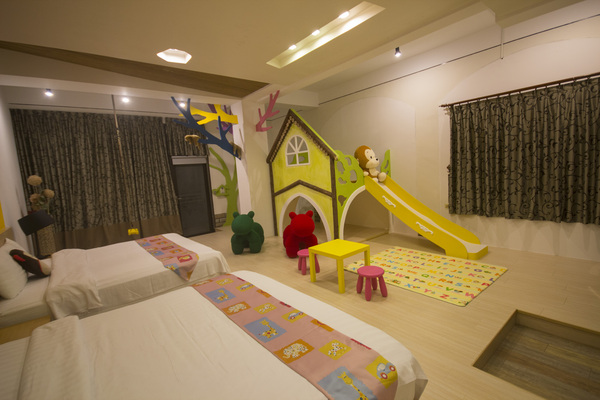客房
•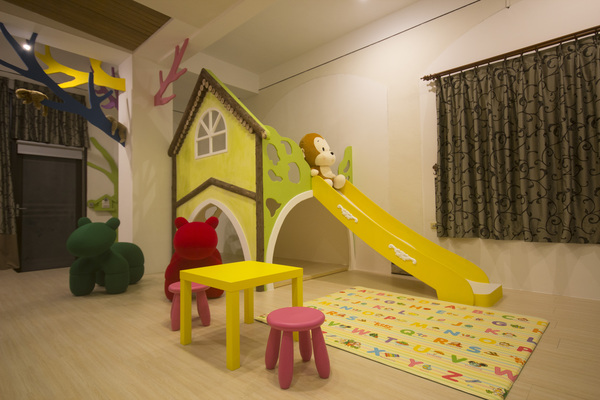客房
•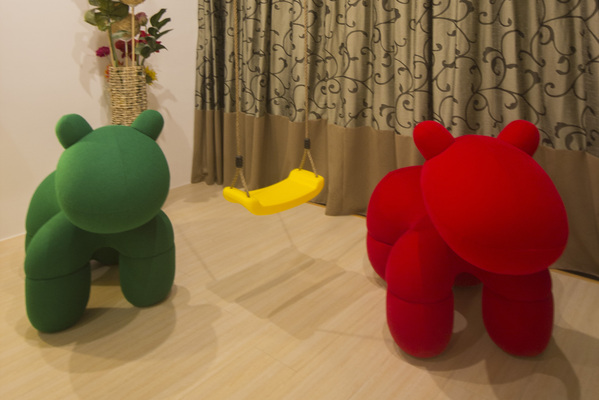客房
•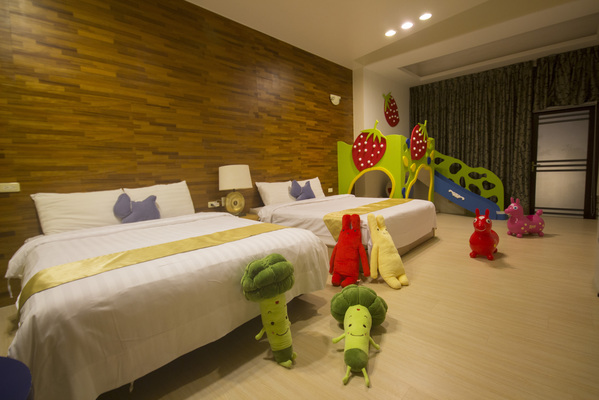客房
•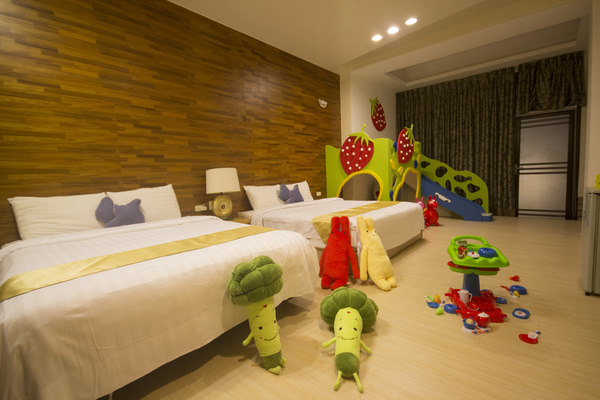客房
•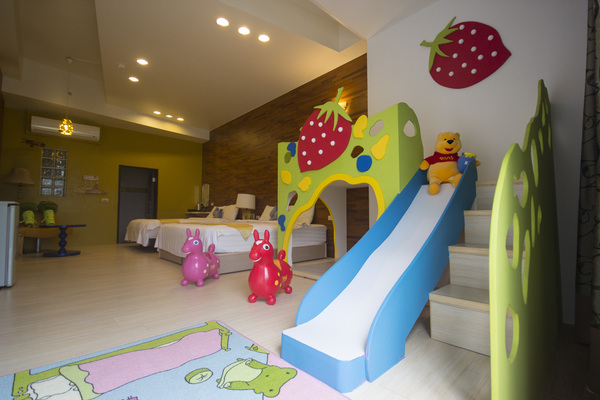客房
•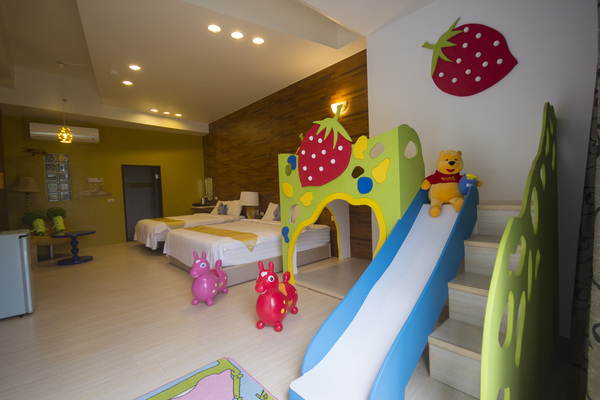客房
•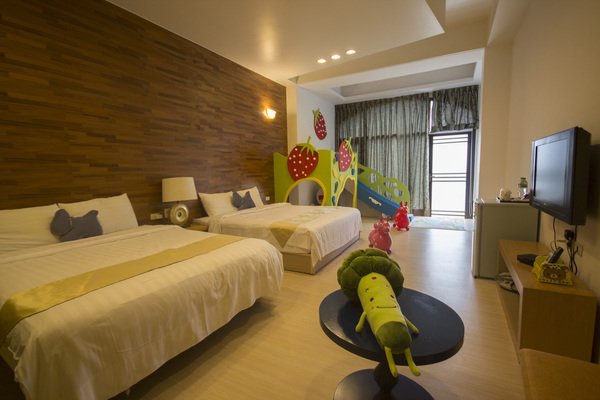客房
•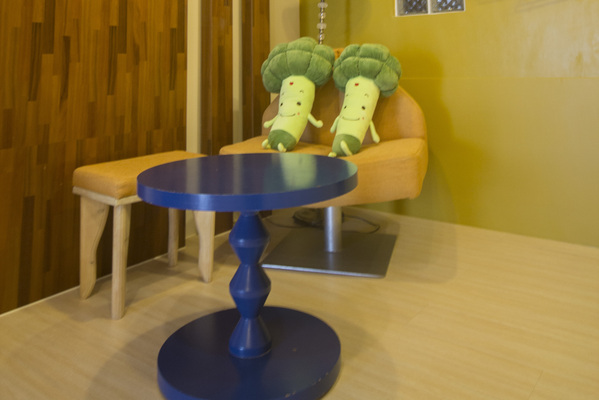客房
•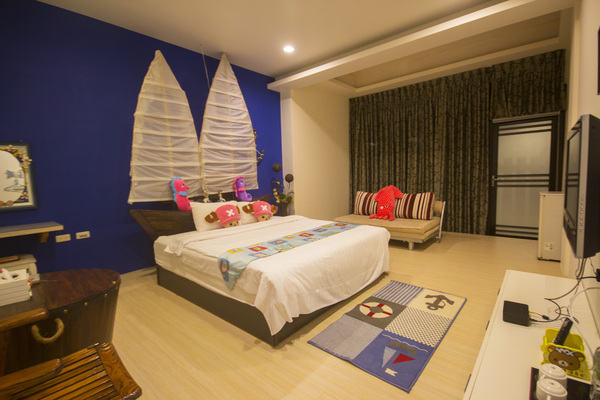客房
•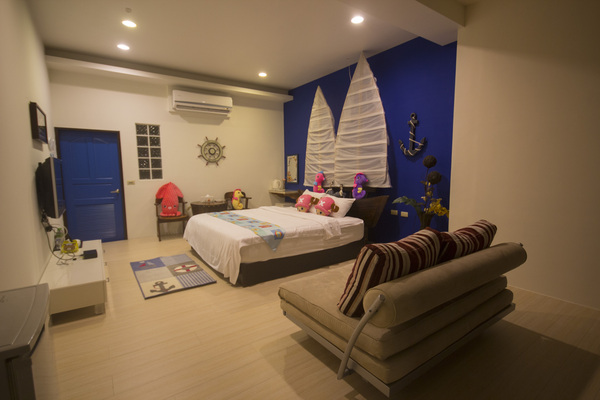客房
•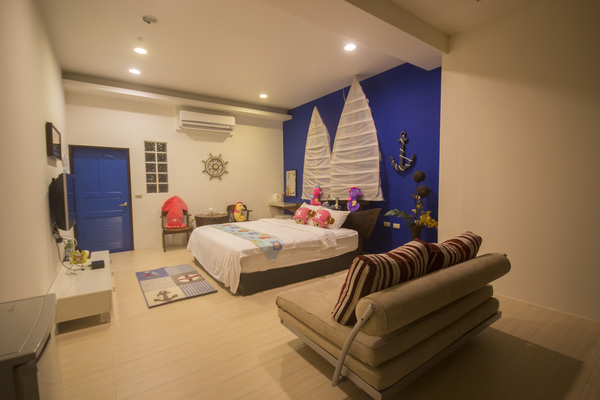客房
•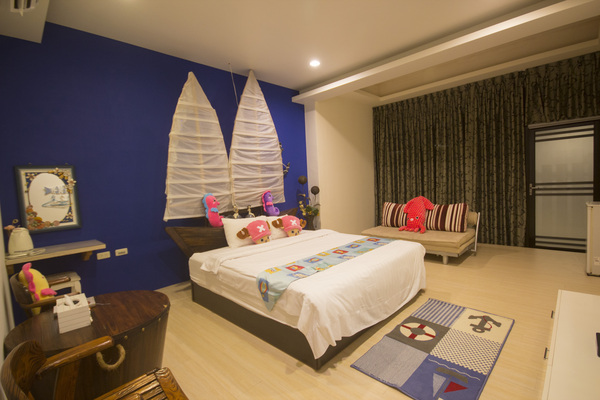客房
•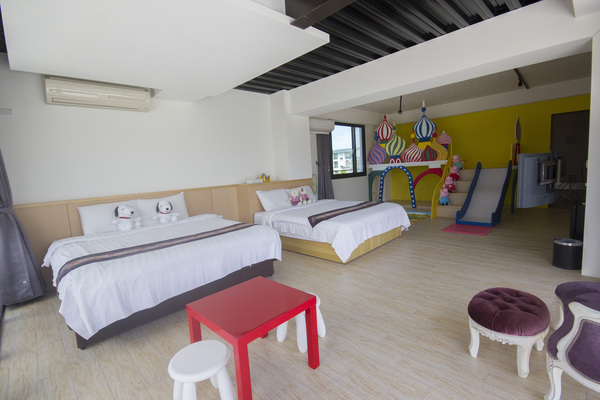客房
•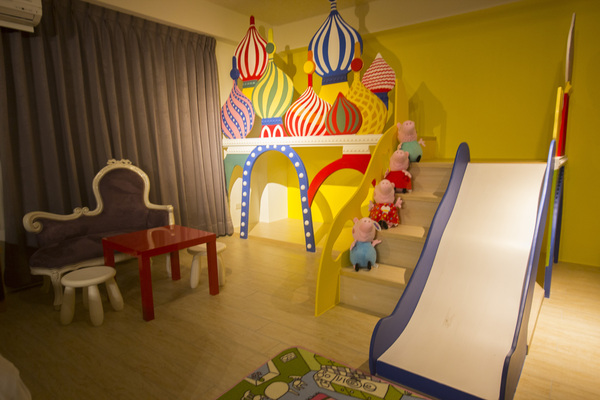客房
•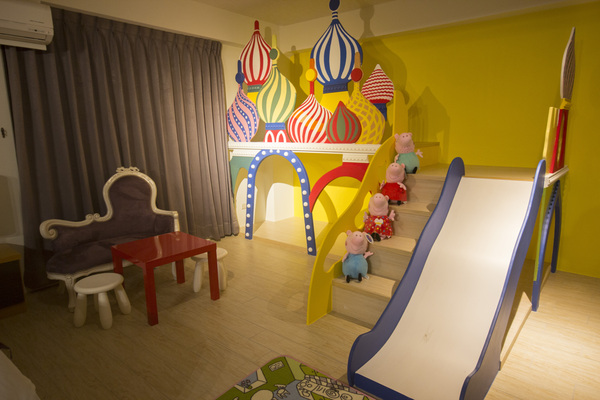客房
•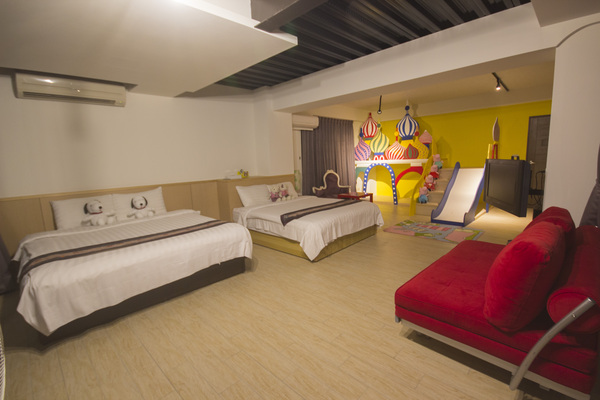客房
•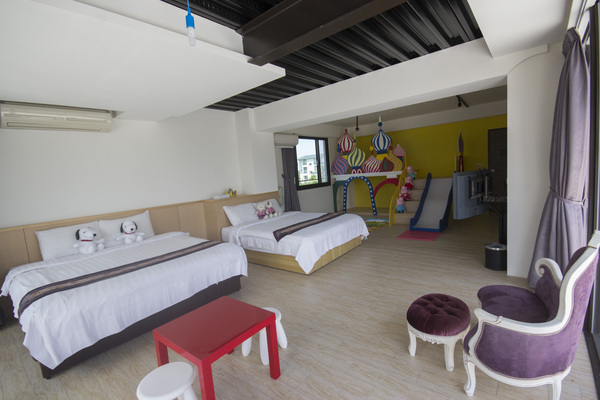客房
•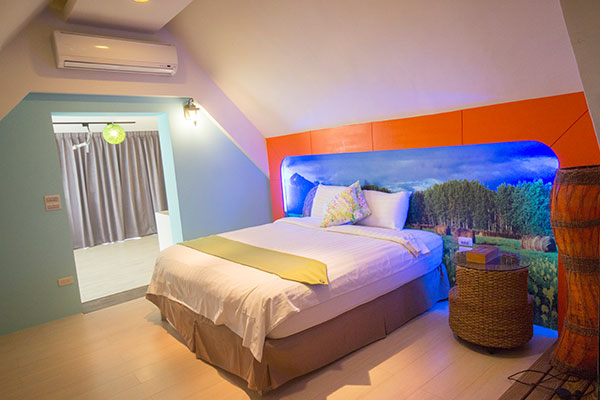客房
•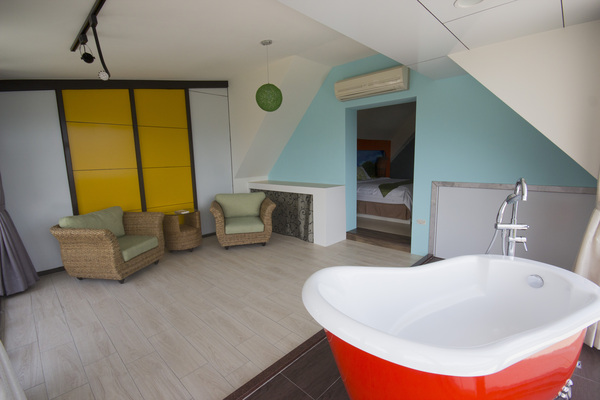客房
•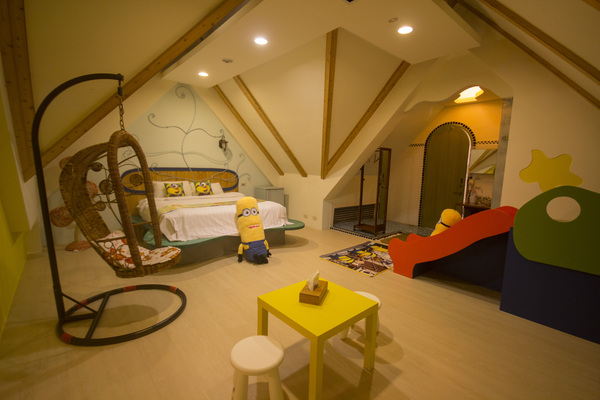客房
•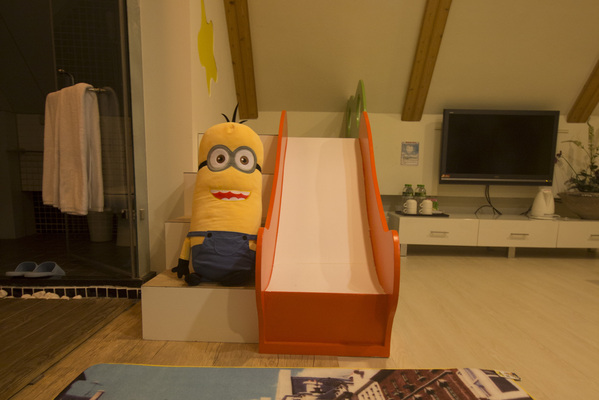客房
•客房
•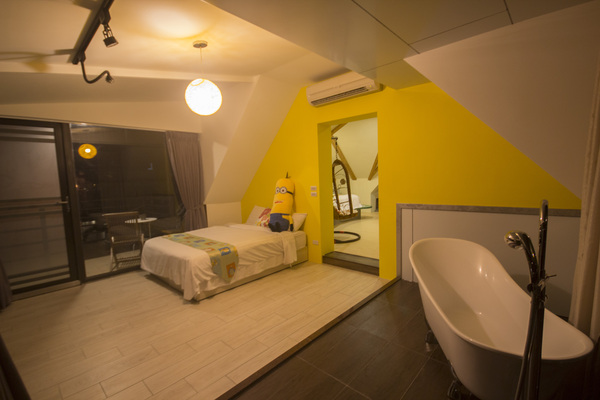客房
•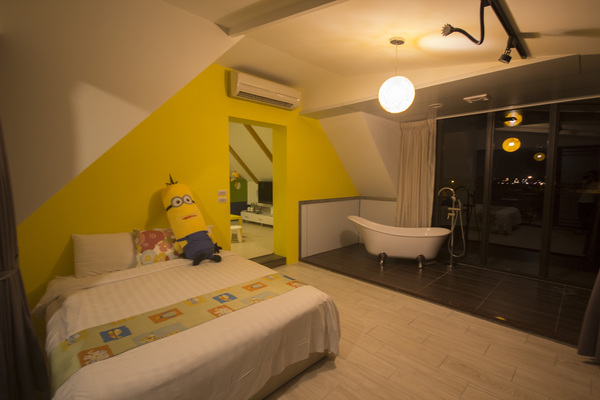客房
•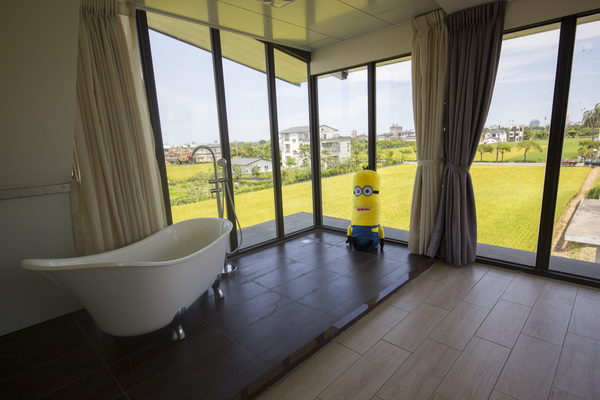客房

#### 飯店設有　 飯店未設 飯店設施

飯店設施
• 24小時客房服務
• 商務中心
• 洗衣服務/乾洗
• Wi-Fi in public areas
• 夜總會
• 禮賓接待服務
• 代客泊車
• 娛樂場
• 自行車出租服務
• 保管服務
• 客房服務
• 行政樓層
• 停車場
• 家庭房
• 酒吧
• 兒童看護服務
• 接送服務
• 電梯
• 可帶寵物
• 旅遊服務
• 飯店/機場接送
• 吸煙房
• 會議設施
• 餐廳
• 咖啡店
• 殘障人士設施
• 髮廊
• 商店
• 池畔吧
房間設施
• DVD/CD播放器
• 小廚房
• 無線上網(收費)
• 休憩區
• 微波爐
• 燙衣板
• 免費瓶裝水
• 房內保險箱
• 獨立淋浴間
• 公共浴室
• 房內遊戲機
• 獨立用餐區
• 冰箱
• 手提電腦保險箱
• 禁煙房
• 冷氣
• 按摩浴缸
• 私人泳池
• 加長的床(>2m)
• 日報
• 茶與咖啡沖泡設備
• 可提供相連房間
• 書桌
• 衛星頻道/有線電視
• 吹風機
• 洗衣機
• 迷你吧
• 寬頻上網
• 浴室
• 陽台/露台
• 寬頻上網(免費)
• 浴缸
• 電影點播服務
• 寬頻上網(收費)
• 浴袍
• 電視
• 寬頻上網(無線)
• 液晶電視
• 電風扇
• 尊用休息室
• 無線上網(免費)
運動&娛樂設施
• spa
• 三溫暖/水療設備
• 網球場
• 健身房/健身設施
• 水上活動
• 花園
• 兒童娛樂室
• 水上運動項目(非機動項目)
• 蒸浴室
• 壁球場
• 溫泉
• 遊戲室/娛樂場
• 室內游泳池
• 游泳池(兒童)
• 高爾夫球場(3km內)
• 室外游泳池
• 漩渦按摩浴池
• 高爾夫球場(飯店)
• 按摩
• 私人海灘

#### 飯店簡介

```古意是我們家族遺傳的個性，我們想保留這個特點，而又希望好朋友們能感受到更多元化的氛圍，因此我們選擇了後現代的建築觀點，將YUDO以「現代日式風格」來設計規劃。兩年的時間，我們的家終於完成了，每個角落都有我們的小故事，當然，古意的我們仍然古意…

交通資訊
◎火車:坐到羅東火車站
◎客運:坐到羅東轉運站```

#### 飯店條款

兒童及加床需求：
• 加床政策根據您所選定的客房而有所不同，更多詳情請查看您所選定的客房的政策

#### 相關資訊

退房時間 11:00 AM 3:00 PM
縮小搜尋結果範圍
0
0